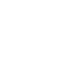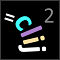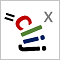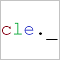# CLIJ2GPU accelerated image processing for everyone

CLIJ2 home

## binarySubtractSubtracts one binary image from another.

### Parameters

minuend : Image The first binary input image to be processed. suubtrahend : Image The second binary input image to be subtracted from the first. destination : Image The output image where results are written into.

Categories: Math, Binary

Availability: Available in Fiji by activating the update sites clij and clij2. This function is part of clij2_-2.5.0.1.jar.

### Usage in ImageJ macro

``````Ext.CLIJ2_binarySubtract(Image minuend, Image subtrahend, Image destination);
``````

### Usage in object oriented programming languages

Java
```// init CLIJ and GPU
import net.haesleinhuepf.clij2.CLIJ2;
import net.haesleinhuepf.clij.clearcl.ClearCLBuffer;
CLIJ2 clij2 = CLIJ2.getInstance();

// get input parameters
ClearCLBuffer minuend = clij2.push(minuendImagePlus);
ClearCLBuffer subtrahend = clij2.push(subtrahendImagePlus);
destination = clij2.create(minuend);
```
```// Execute operation on GPU
clij2.binarySubtract(minuend, subtrahend, destination);
```
```// show result
destinationImagePlus = clij2.pull(destination);
destinationImagePlus.show();

// cleanup memory on GPU
clij2.release(minuend);
clij2.release(subtrahend);
clij2.release(destination);
```
Matlab
```% init CLIJ and GPU
clij2 = init_clatlab();

% get input parameters
minuend = clij2.pushMat(minuend_matrix);
subtrahend = clij2.pushMat(subtrahend_matrix);
destination = clij2.create(minuend);
```
```% Execute operation on GPU
clij2.binarySubtract(minuend, subtrahend, destination);
```
```% show result
destination = clij2.pullMat(destination)

% cleanup memory on GPU
clij2.release(minuend);
clij2.release(subtrahend);
clij2.release(destination);
```
Icy JavaScript
```// init CLIJ and GPU
importClass(net.haesleinhuepf.clicy.CLICY);
importClass(Packages.icy.main.Icy);

clij2 = CLICY.getInstance();

// get input parameters
minuend_sequence = getSequence();
minuend = clij2.pushSequence(minuend_sequence);
subtrahend_sequence = getSequence();
subtrahend = clij2.pushSequence(subtrahend_sequence);
destination = clij2.create(minuend);
```
```// Execute operation on GPU
clij2.binarySubtract(minuend, subtrahend, destination);
```
```// show result
destination_sequence = clij2.pullSequence(destination)
```import pyclesperanto_prototype as cle